# Module number and module properties

## The module definition

Definition: a Module is a positive number called the number, modulus of a negative number is a number, its opposite, module zero is zero.

### Examples of location module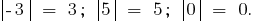## The geometric meaning of the module

Set cut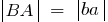.Definition: On the coordinate axis module is the distance from the origin to the point representing the given number.

Definition: the absolute difference of two numbers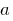i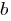is the distance between pointsandon the coordinate axis.

## Module properties

1.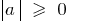(Modulus of any number is non — negative)
2.(Modules of opposite numbers are equal)
3.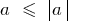(The amount doesn't exceed the size of the module)
4.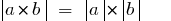(Module works dorwn the product of the moduli of the factors)
5.(A unit fraction is equal to the modulus of the numerator divided by the module of the denominator (if the denominator is not zero))
6.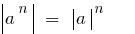7.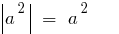8.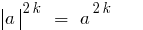9.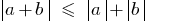(Module does not exceed the amount of the sum of absolute terms)
10.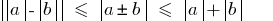Tags:
Chapter:
Versions in other languages:
Share with friends: